You can be sure that you’re going to encounter exponents on the SAT. Hopefully, once you’ve been through this post, you’ll look forward to the opportunity to spank these questions like they deserve. Look at them, mocking you. They’re totally asking for it. Show no mercy.

##### The Basic Exponent Rules
• Anything to the first power equals itself:
y1 = y
• Anything raised to the zero power equals 1:
m0 = 1
• Remember that a regular old integer exponent means something very simple:
x4 = xxxx. Don’t be ashamed to do this when you’re stuck. You’ll be amazed how often this will unstick you.
• When you multiply like bases, you add their exponents:
p3p5 = p3+5 = p8. Why? See the bullet above.
• When you divide like bases, you subtract the exponents:
r9/r4 = r9-4 = r5. Again, why is this true? Think about it. Does it blow your mind?
• When you raise an exponent to another power, you multiply the exponents:
(z3)7 = z21. Why is that?
• When you’re adding or subtracting like bases, you can’t do JACK:
y3 + y4y3y4. There’s no simplifying (although you might decide to factor a y3 out depending on where you’re trying to go — remember this for practice problem #18 below). If you make the mistake of adding these exponents, people will laugh at you in public places.

Before we go further, let’s make sure you’ve got this. Try simplifying the following. Hold your mouse over the questions when you’re done, and the answers will reveal themselves, as though they were genies and you Aladdin. Don’t click, just hover.

• t9t2 =
• 3n/32 =
• (xy3)(x5y) =
• (8m)2 =
• r16r12 =
##### Fractional and Negative Exponents

Honestly, these appear very rarely on the SAT. I’m including them for completeness’s sake, but don’t sweat these too hard.

• Fractional exponents (to convert the exponent to radical form):
• The numerator is the power.
• The denominator is the root.
• In other words: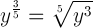• Negative exponents
• A negative exponent signifies the reciprocal of the corresponding positive exponent.
• In other words, the base is on the wrong side of the fraction line.
• In other words: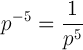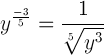What’s nice is that even though these rules might be a bit more confusing, fractional and negative exponents follow the same rules as integer exponents. Stare at these for a minute to see what I mean: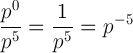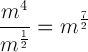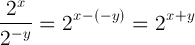##### Try some practice questions now, bro!

You need to be registered and logged in to take this quiz. Log in or RegisterChloe M R says:

wait how do you do the last question??Mike McClenathan says:

Tricky, no? The key to this question is factoring. Take 2^499 out of (2^500 – 2^499):

(2^500 – 2^499) = (2^499)(2 – 1) = 2^499

You can, of course, do the same with the other part of the expression:

(2^400 – 2^399) = (2^399)(2 – 1) = 2^399

So basically, the whole business simplifies to:

(2^499)(2^399) = 2^898MH says:

Help on #17!Mike McClenathan says:

Plugging in works really well here. Say z = 2, p = 3, and q = 5. Only one answer should work.

If you’d rather use the rules, then you need to try to manipulate every choice until one simplifies to z^q. (D) works because you can simplify it like this:

(z^p)/(z^(pq)) ← Start
z^(p – (pq)) ← Same base division means subtract exponents
z^(pp + q) ← Distribute the negative
z^qJoe Lanzone says:

Why doesn’t C work too?Mike McClenathan says:

If you apply the exponent rule to (C), it simplifies to z^(pq – p). That’s not the same as z^q. Plug in to convince yourself. Say p = 3 and q = 5. z^(pq – p) = z^(15 – 5) = z^10. But z^q is just z^5. Not the same thing.Joe Lanzone says:

Thanks!Michael says:

There is a slight error when you say “Anything raised to the zero power equals 1”. For example, 0^0 = undefined, not 1.Mike McClenathan says:

So there you go…disagreement. Sometimes that happens, even in math. But don’t worry about the SAT, I can tell you with certainty that you won’t encounter 0^0 there.henry says:

(8m)2

Wouldn’t this be 64^2m ?Mike McClenathan says:

Nope…it’s (8^m)(8^m) = 8^(m+m) = 8^(2m)henry says:

Thanks for clarifying. I was under the assumption that everything gets squared because it was inside the parentheses. It looks like I have work to do on exponents, but thankfully I have this!Katie says:

how is m^4/m^1/2= m^7/2?Mike McClenathan says:

See the attached image 🙂Katie says:

For #17 I’m confused because I plug in z=2, p=3 and q=5, and when i do the work i get 2^3/2^-1.., how does that come out to 8? it comes out to 16?Mike McClenathan says:

I think you’re getting turned around here with the p and the q. You’re looking for the choice that’s equal to z^q, which with your numbers should be 2^5 = 32. So you have (2^3)/(2^(3–5)) = (2^3)/(2^–2), which does indeed equal 32.GURMANNAT SINGH says:

SUPPOSE :
4^X IS DIVIDED BY 8^Y
What is the value of X by YMike McClenathan says:

4^x = 2^(2x)
8^y = 2^(3y)

So 4^x / 8^y = 2^(2x)/2^(3y) = 2^(2x – 3y)